# 填充每个节点的下一个右侧节点指针

## 题目

struct Node {
int val;
Node *left;
Node *right;
Node *next;
}


• 你只能使用常量级额外空间。

### 例子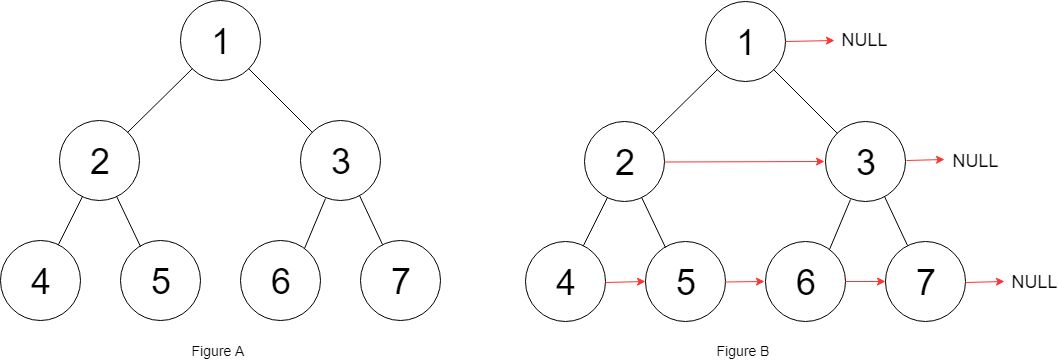输入：root = [1,2,3,4,5,6,7]



• 树中节点的数量少于 4096

• -1000 <= node.val <= 1000

（1）如果有右侧节点

（2）如果没有右侧节点

## java 实现

public Node connect(Node root) {
List<List<Node>> results = new ArrayList<>();
levelOrder(results, root, 0);
return root;
}

private void levelOrder(List<List<Node>> results, Node node, int level) {
if(node == null) {
return;
}
// 当前节点
// AVOID BOUND EX
if(results.size() <= level) {
}
List<Node> list = results.get(level);
// 如果列表不为空，把上一个 node 的 next 指向当前 Node
if(list.size() > 0) {
Node pre = list.get(list.size()-1);
pre.next = node;
}
// 当前层最后一个元素
int maxNum = (int) Math.pow(2, level);
if(list.size() == maxNum-1) {
node.next = null;
}
// 节点
results.set(level, list);
// 左
levelOrder(results, node.left, level+1);
// 右
levelOrder(results, node.right, level+1);
}


Runtime: 1 ms, faster than 57.79% of Java online submissions for Populating Next Right Pointers in Each Node.
Memory Usage: 39.2 MB, less than 70.19% of Java online submissions for Populating Next Right Pointers in Each Node.


## 优化思路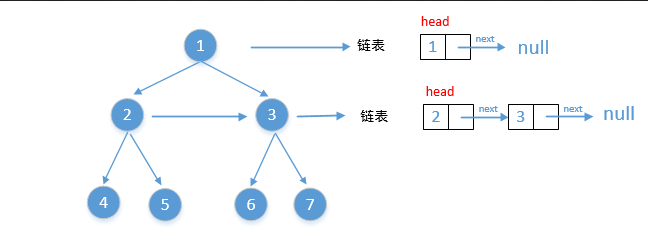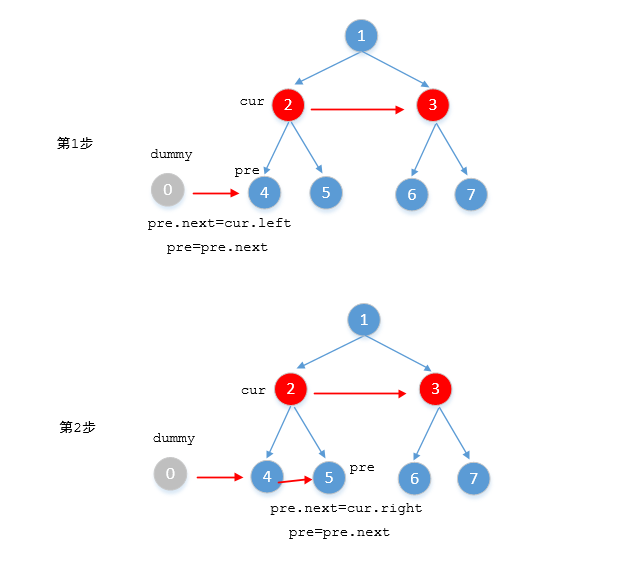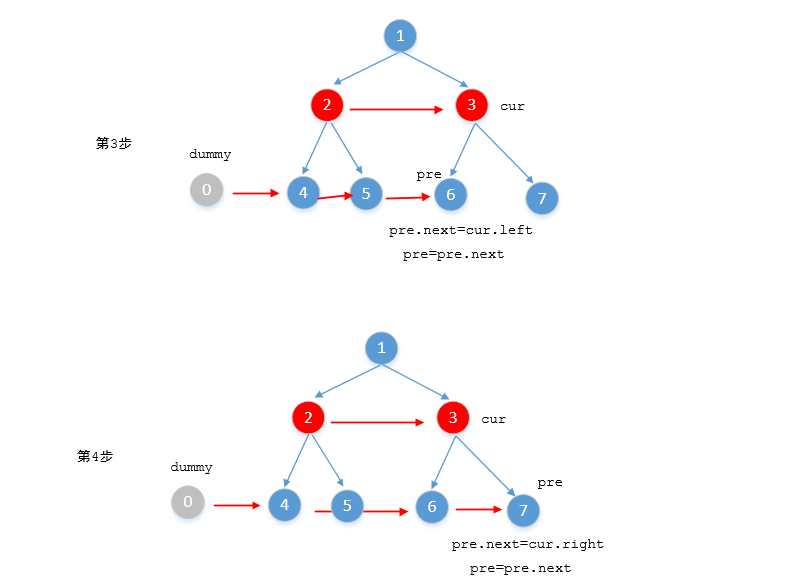（1）左子树

（2）右子树

## java 实现

public Node connect(Node root) {
connect(root, null);
return root;
}

private void connect(Node current, Node next) {
// 终止条件
if (current == null) {
return;
}
// 核心逻辑
current.next = next;

// 左=》右（当前节点，左子树=》右子树）
connect(current.left, current.right);
// 右子树，指向当前节点 next 的左子树，或者指向空
connect(current.right, current.next == null ? null : current.next.left);
}


Runtime: 0 ms, faster than 100.00% of Java online submissions for Populating Next Right Pointers in Each Node.
Memory Usage: 39.3 MB, less than 45.38% of Java online submissions for Populating Next Right Pointers in Each Node.


# 填充每个节点的下一个右侧节点指针 II

## 题目

struct Node {
int val;
Node *left;
Node *right;
Node *next;
}


### 例子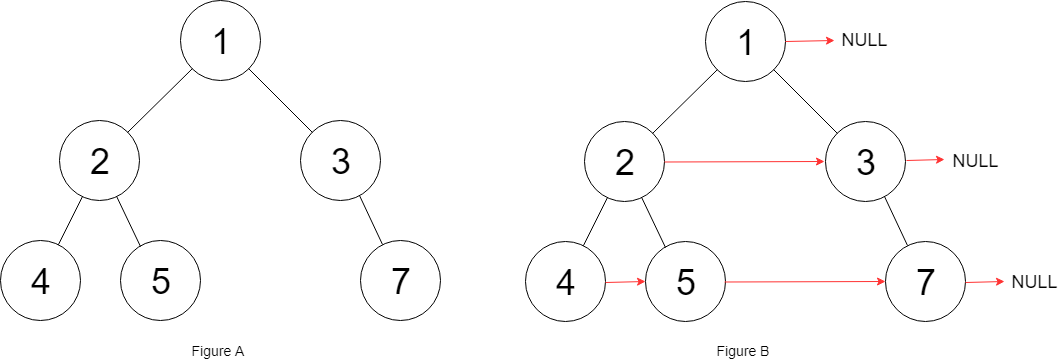输入：root = [1,2,3,4,5,null,7]



• 树中的节点数小于 6000

• -100 <= node.val <= 100

## 思路 1

（1）层序遍历获取所有元素

（2）按照层级，依次设置 next 指向

### java 实现

public Node connect(Node root) {
List<List<Node>> results = new ArrayList<>();
connect(results, root, 0);
// 设置 next
for(int i = 0; i < results.size(); i++) {
List<Node> list = results.get(i);
for(int j = 1; j < list.size(); j++) {
Node pre = list.get(j-1);
pre.next = list.get(j);
}
}
return root;
}

private void connect(List<List<Node>> results, Node node, int level) {
if (node == null) {
return;
}
// AVOID BOUND EX
if(results.size() <= level) {
}
List<Node> list = results.get(level);
if(list == null) {
list = new ArrayList<>();
}
results.set(level, list);
connect(results, node.left, level+1);
connect(results, node.right, level+1);
}


Runtime: 1 ms, faster than 55.82% of Java online submissions for Populating Next Right Pointers in Each Node II.
Memory Usage: 38.7 MB, less than 50.24% of Java online submissions for Populating Next Right Pointers in Each Node II.


## 思路 2

### java 实现

// 上一个节点
private Node pre = null;
// 下一层的开始节点
private Node nextStart = null;
public Node connect(Node root) {
Node start = root;
while (start != null) {
pre = null;
nextStart = null;
Node current = start;
while (current != null) {
// 处理下一层的 next 关系
handle(current.left);
handle(current.right);
// 移动当前层的位置
current = current.next;
}
// 下一层的开始节点
start = nextStart;
}
return root;
}

private void handle(Node current) {
if(current == null) {
return;
}
// 设置子节点层 pre.next = current
if (pre != null) {
pre.next = current;
}
// 更新 pre
pre = current;
// 设置下一层的开始节点（第一个非空的元素）
if (nextStart == null) {
nextStart = current;
}
}


Runtime: 0 ms, faster than 100.00% of Java online submissions for Populating Next Right Pointers in Each Node II.
Memory Usage: 38.9 MB, less than 29.02% of Java online submissions for Populating Next Right Pointers in Each Node II.


# 参考资料

https://leetcode-cn.com/problems/populating-next-right-pointers-in-each-node/